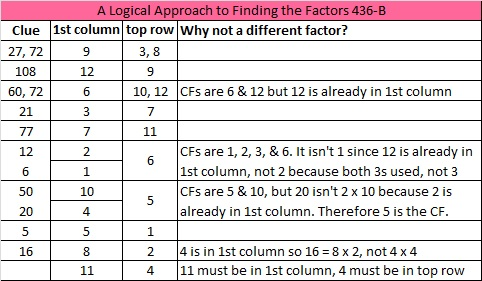# 230 and Level 5

• 230 is a composite number.
• Prime factorization: 230 = 2 x 5 x 23
• The exponents in the prime factorization are 1, 1, and 1. Adding one to each and multiplying we get (1 + 1)(1 + 1)(1 + 1) = 2 x 2 x 2 = 8. Therefore 230 has 8 factors.
• Factors of 230: 1, 2, 5, 10, 23, 46, 115, 230
• Factor pairs: 230 = 1 x 230, 2 x 115, 5 x 46, or 10 x 23
• 230 has no square factors so its square root cannot be simplified. √230 ≈ 15.166Excel file of puzzles and previous week’s factor solutions: 12 Factors 2014-09-08